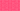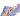PricingResources

# How to Calculate avg rate of return (ARR)

Written by

There are multiple metrics to consider before jumping into any investment, one of which is the average rate of return (ARR). Average rate of return offers a simple way to calculate an investment’s potential for profit or risk. In this guide, we’ll cover how to calculate ARR as well as what to do with this information.

## What is ARR in accounting?

This acronym stands for either accounting rate of return or average rate of return, both referring to an investment’s expected rate of return in comparison to its initial cost. This capital budgeting formula evaluates the long-term profit potential for any investment by weighing up average yearly revenue with start-up costs.

Calculating ARR revenue is a good idea if you’re considering whether to invest in an expensive piece of machinery or equipment, or whether a new project will pay off in the long run.

While ARR doesn’t give you a completely accurate figure, it can provide a basic snapshot of any investment’s potential earnings over time. It’s related to the required rate of return (RRR), but while RRR gives a minimum threshold of profit, ARR is more flexible.

## What is the average rate of return (ARR) formula?

To calculate ARR revenue as a percentage, you must take the asset’s average yearly revenue and divide by initial cost.

The ARR formula looks like this:

This will give you a decimal figure, which is then multiplied by 100 to create a percentage.

## How to calculate ARR

Calculating ARR is simple once you’ve determined the figures for annual profit and investment costs. Here’s a step-by-step breakdown:

1. Determine average annual profit for the investment by taking the total projected profit and dividing it by the number of years the investment will be producing profit. This should be a net profit, or revenue remaining after deducting all associated taxes, expenses, and other related costs.

2. If you’re investing in a fixed asset like property or equipment, you’ll also need to subtract the cost of depreciation.

3. Divide the average annual profit by the investment or asset’s initial cost.

4. Multiply the resulting decimal figure by 100 to see ARR in a percentage format.

There are multiple online calculators that can take care of these calculations on your behalf, or you can seek advice from a financial advisor to work out the likely annual profit.

For an example of how this works, imagine that we’re calculating the average rate of return for an investment property.

• Step 1: The asset’s net present value is \$300,000 and has generated \$200,000 in revenue. To calculate the ARR for an investment period of one year, you would divide the total revenue of \$200,000 by 1 to equal \$200,000.

• Step 2: Imagine that the property is expected to depreciate by \$50,000 in value during the year, which must be subtracted from the annual profit for a total of \$150,000.

• Step 3: Divide the annual net profit of \$150,000 by the initial cost of \$300,000 for purchasing the property. 150,000/300,000 = 0.5

• Step 4: Multiple 0.5 by 100 to equal a 50% average rate of return for the investment’s first year.

## What does ARR mean for a business?

Whether it’s purchasing property, investing in a new software training program, or expanding into a new market, there are many investment decisions that businesses must make. Learning how to calculate ARR helps give you some idea of whether the investment is worth the time, cost, and effort. These calculations are also useful when comparing multiple investments because you can see which will be the most profitable. The result is a more informed decision-making process for growth.

#### We can help

GoCardless helps you automate payment collection, cutting down on the amount of admin your team needs to deal with when chasing invoices. Find out how GoCardless can help you with ad hoc payments or recurring payments.## Interested in automating the way you get paid? GoCardless can help

Contact sales

Sales

Contact Sales

+1(415) 523-2279

Support

help@gocardless.com

+1 (628) 241-0044